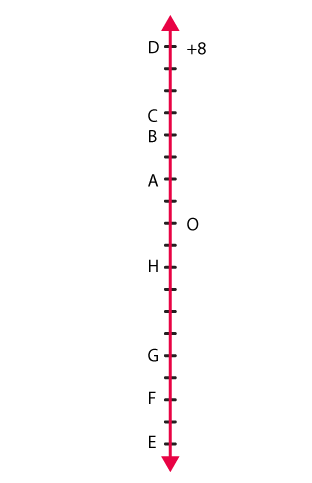# Adjacent figure is a vertical number line, representing integers. Observe it and locate the following points: (a) If point D is + 8, then which point is - 8? (b) Is point G a negative integer or a positive integer? (c) Write integers for points B and E. (d) Which point marked on this number line has the least value? (e) Arrange all the points in decreasing order of value.Solution:

(a)If point D is +8, then point F is -8

(b) Point G is a negative integer

(c) Point B is 4 and point E is – 10

(d) The least value on this number line is point E as it represents – 10

(e) The points in decreasing order of value are D, C, B, A, O, H, G, F, E(0)(0)